﻿ 连续管钻井循环压耗的计算 The Calculation of Circulating Pressure Loss in Coiled-tubing Drilling

Journal of Oil and Gas Technology
Vol.39 No.06(2017), Article ID:23060,12 pages
10.12677/JOGT.2017.396100

The Calculation of Circulating Pressure Loss in Coiled-tubing Drilling

Yuanzhu Mi, Yue Luo, Zhiming Huang

School of Chemistry & Environmental Engineering, Yangtze University, Jingzhou Hubei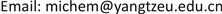Received: Oct. 30th, 2017; accepted: Nov. 7th, 2017; published: Dec. 15th, 2017ABSTRACT

A block of Liaohe Oilfield was taken as research object, based on geological condition of the block and performance parameter of coiled-tubing drilling rig, the pressure loss of different sections was calculated in coiled-tubing drilling(CT) including pressure loss of CT twisting in reel, downhole CT, annulus and total circulating. Especially, the effect of some important factors on total circulating pressure loss was discussed such as flow rate Q, consistency index K and rheological index n. Rheological properties parameter for coiled drilling fluid was determined by combining calculation results and practical experience. The calculation results show that it was desirable that Q is selected at 0.01 m3/s, n is at 0.4-0.5 and K is at 0.7-1.0 Pa∙sn in the water-based solid-free drilling fluid. Certain theoretical basis is provided for the design of fluids used in coiled-tubing drilling.

Keywords:Coiled-tubing Drilling, Water-based Drilling Fluid, Circulating Pressure Loss, Rheological Property Parameter1. 引言

2. 连续管钻井循环压耗计算公式

2.1. 连续管直管循环压耗计算公式

$Re=\frac{\rho {d}^{n}{\nu }^{2-n}}{{8}^{n-1}K{\left(\frac{3n+1}{4n}\right)}^{n}}$ (1)

${f}_{C}=\frac{16}{Re}$ (2)

${f}_{W}=\frac{a}{R{e}^{b}}$ (3)

$a=\frac{\mathrm{lg}n+3.93}{50}$ (4)

$b=\frac{1.75-\mathrm{lg}n}{7}$ (5)

${f}_{G}=\frac{16}{{C}_{1}}+\left(\frac{a}{{C}_{2}^{b}}+\frac{16}{{C}_{1}}\right)\frac{Re-{C}_{1}}{800}$

$\begin{array}{l}{C}_{1}=3470-1370n\\ {C}_{2}=4270-1370n\end{array}$ (6)

$\Delta p=\frac{2\rho {\nu }^{2}}{d}{f}_{j}L$ (7)

2.2. 环空中循环压耗的计算公式

$Re=\frac{\rho {\left({D}_{2}-{D}_{1}\right)}^{n}{\nu }^{2-n}}{{12}^{n-1}K{\left(\frac{2n+1}{3n}\right)}^{n}}$ (8)

${f}_{HC}=\frac{24}{Re}$ (9)

${f}_{HW}=\frac{a}{R{e}^{b}}$ (10)

$a=\frac{\mathrm{lg}n+3.93}{50}$ (11)

$b=\frac{1.75-\mathrm{lg}n}{7}$ (12)

${f}_{HG}=\frac{24}{{C}_{1}}+\left(\frac{a}{{C}_{2}^{b}}+\frac{24}{{C}_{1}}\right)\frac{Re-{C}_{1}}{800}$ (13)

$\begin{array}{l}{C}_{1}=3470-1370n\\ {C}_{2}=4270-1370n\end{array}$ (14)

$\Delta p=\frac{2\rho {\nu }^{2}}{{D}_{2}-{D}_{1}}{f}_{Hj}L\text{\hspace{0.17em}}\text{\hspace{0.17em}}\text{\hspace{0.17em}}\left(\text{j}=\text{C},\text{W},\text{G}\right)$ (15)

2.3. 盘管中循环压耗的计算公式

${N}_{Dn}=Re×{\left[\frac{{r}_{0}}{{R}_{i}}\right]}^{0.5}$ (16)

${f}_{PC}=\frac{16}{{N}_{Dn}}$ (17)

${f}_{PW}=\frac{a}{{N}_{Dn}^{b}}$ (18)

$a=\frac{\mathrm{lg}n+3.93}{50}$ (19)

$b=\frac{1.75-\mathrm{lg}n}{7}$ (20)

${f}_{PG}=\frac{16}{{C}_{1}}+\left(\frac{a}{{C}_{2}^{b}}+\frac{12}{{C}_{1}}\right)\frac{{N}_{Dn}-{C}_{1}}{800}$ (21)

$\begin{array}{l}{C}_{1}=3470-1370n\\ {C}_{2}=4270-1370n\end{array}$ (22)

$\Delta p=\frac{2\rho {\nu }^{2}}{d}\sum _{i=1}^{N}{f}_{j}L\text{\hspace{0.17em}}\text{\hspace{0.17em}}\text{\hspace{0.17em}}\left(\text{j}=\text{C},\text{W},\text{G}\right)$ (23)

2.4. 连续管钻井循环压耗计算参数

3. 连续管钻井循环压耗的计算

3.1. Q值对循环压耗的影响

${R}_{i}=\frac{\sqrt{{\left[\left(2{r}_{0}+B\right)+2\sqrt{3}\left(i-1\right){r}_{0}\right]}^{2}+{r}_{0}^{2}}}{2}\text{\hspace{0.17em}}\text{\hspace{0.17em}}\text{\hspace{0.17em}}\left(N\ge i\ge 1\right)$ (24)

${L}_{p}=2\pi M\sum _{i=1}^{N}{R}_{i}$ (25)

${L}_{i}=2\pi M{R}_{i}$ (26)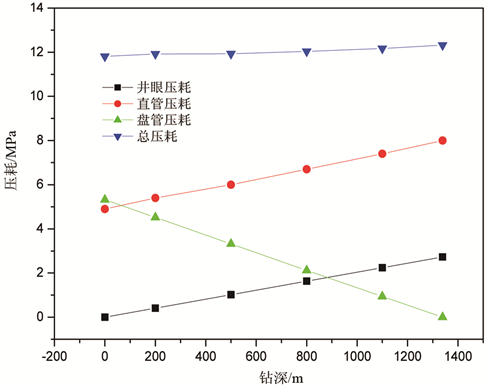Figure 1. The pressure loss in each section at different drilling depths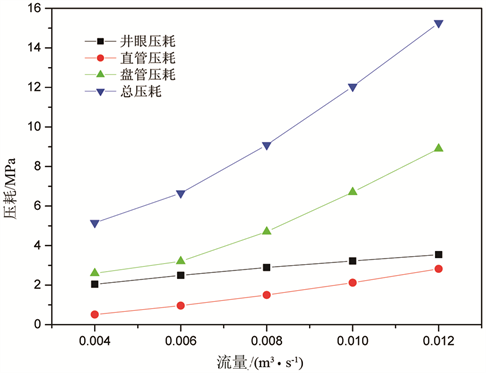Figure 2. The effect of flow rate on the pressure loss in each section at 800 m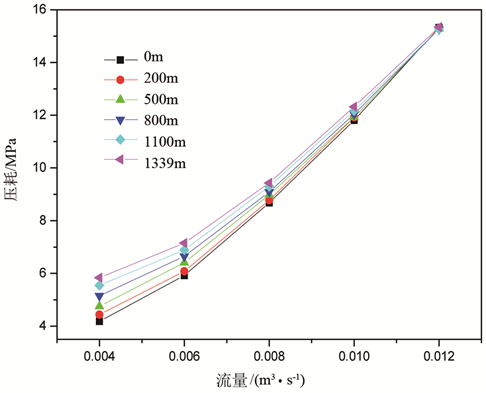Figure 3. The effect of Q on the total circulating pressure loss at different drilling depths

3.2. n值对循环压耗的影响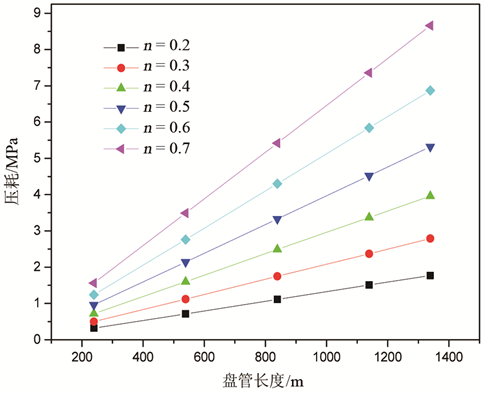Figure 4. The effect of n on the pressure loss of each section of CT twisting in reel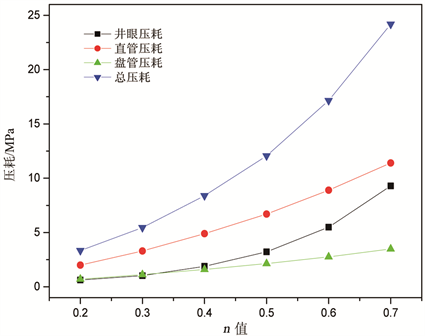Figure 5. The effect of n on the pressure loss of each section at 800 m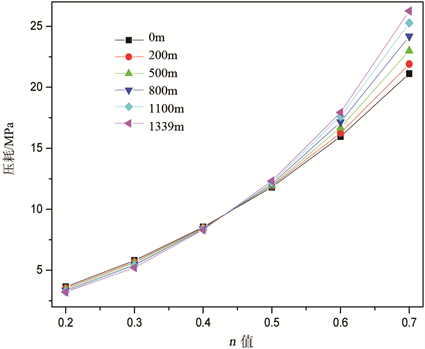Figure 6. The effect of n on the total circulating pressure loss at different drilling depths

3.3. K值对循环压耗的影响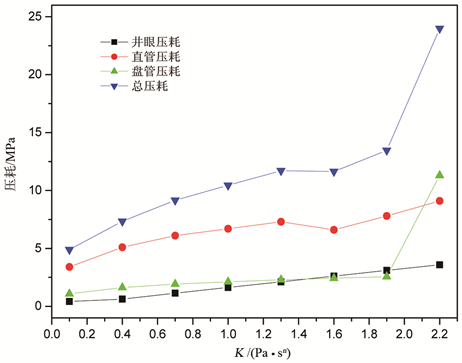Figure 7. The effect of K on the pressure loss of each section at 800 m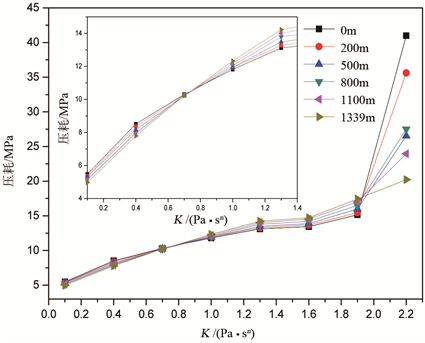Figure 8. The effect of K on the total circulation pressure loss at different drilling depths

4. 结论

1) 各段压耗以及总循环压耗均随着流量Q的增加而增加，但是流量Q对环空压耗和盘管压耗的影响相对较小。对直管段而言，只有当流量大于0.06 m3/s时，对压耗的影响才比较大。考虑到马达驱动、总循环压耗以及压耗稳定，选择0.01 m3/s作为最佳流量。

2) 随着n值的增加，连续管盘管和直管压耗均呈线性增加。当n值较小时，对环空压耗影响较小，但是较大的n值会在环空中产生较大的压耗。总循环压耗随着n值的增加而增加，而且增加的幅度也越大。结合不同钻深时的压耗计算，选择适宜的n值为0.4~0.5。

3) 随着K值的变化，流体经历了3种流型，除了当K值为1.6 Pa∙sn时，由于直管中的流体流型变为层流使直管压耗略有降低，总体而言，随着K值的增大，各段压耗及总循环均增大。当K值大于1.9 Pa∙sn后，由于盘管中的流体流型变为层流，使盘管压耗和总循环均大幅度增加。综合考虑总循环压耗、压耗稳定和钻屑的有效携带，K值宜选择在0.7~1.0 Pa∙sn

The Calculation of Circulating Pressure Loss in Coiled-tubing Drilling[J]. 石油天然气学报, 2017, 39(06): 67-78. http://dx.doi.org/10.12677/JOGT.2017.396100

1. 1. Ian, W.C. and Gu, H. (1996) Hydraulics Design in Coiled Tubing Drilling. SPE36349.

2. 2. McCann, R.C. and Islas, C.G. (1996) Frictional Pressure Loss during Turbulent Flow in Coiled Tubing. SPE36345. https://doi.org/10.2118/36345-MS

3. 3. Azouz, I., Shah, S.N., Vinod, P.S., et al. (1998) Experimental Investigation of Frictional Pressure Losses in Coiled Tubing. Society of Petroleum Engineers Production & Facilities, 13, 91-96. https://doi.org/10.2118/37328-PA

4. 4. Rao, B.N. (2002) Friction Factor for Turbulent Flow of Non-Newtonian Fluid in Coiled Tubing. SPE74847. https://doi.org/10.2118/74847-MS

5. 5. Zhou, Y. and Shah, S.N. (2006) New Friction-Factor Correlations for Non-Newtonian Fluid Flow in Coiled Tubing. SPE77960. https://doi.org/10.2118/77960-PA

6. 6. Bailey, M.B. and Rosine, R.S. (2009) Comparison of Computional Fluid Dynamics of Erosion in Coiled Tubing on Reel-To-Injector Flow Area. SPE121171.

7. 7. Medjani, B. and Shah, S.N. (2000) A New Approach for Predicting Frictional Pressure Losses of Non-Newtonian Fluid in Coiled Tubing. SPE60319. https://doi.org/10.2118/60319-MS

8. 8. Willingham, J.D. and Shah, S.N. (2000) Friction Pressures of Newtonian and Non-Newtonian Fluids in Straight and Reeled Coiled Tubing. SPE60719. https://doi.org/10.2118/60719-MS

9. 9. Shah, S.N. and Zhou, Y. (2001) An Experimental Study of the Effects of Drilling Solids on Frictional Pressure Losses in Coiled Tubing. SPE67191. https://doi.org/10.2118/67191-MS

10. 10. Shah, S., Zhou, Y., Bailey, M., et al. (2009) Correlations to Predict Frictional Pressure Loss of Hydraulic Fracturing Slurry in Coiled Tubing. SPE104253. https://doi.org/10.2118/104253-PA

11. 11. Zhou, Y.X. (2006) Theoretical and Experimental Studies of Power-Law Fluid Flow in Coiled Tubing. The University of Oklahoma, Oklahoma.

12. 12. Hou, X.J., Zheng, H.K. and Zhao, J. (2014) Analysis of Circulating System Frictional Pressure Loss in Microhole Drilling with Coiled Tubing. The Open Petroleum Engineering Journal, 7, 22-28.

13. 13. Dean, W.R. (1927) Note on the Motion of Fluid in a Curved Pipe. Philosophical Magazine, 4, 208-223. https://doi.org/10.1080/14786440708564324

14. 14. Dean, W.R. (1928) Fluid Motion in a Curved Channel. Proceedings of the Royal Society of London: Series A. Containing Papers of a Mathematical and Physical Character, 121, 402-420. https://doi.org/10.1098/rspa.1928.0205

15. 15. Hou, X.J., Gao, D.L. and Shen, Z.H. (2013) An Analysis of the Flow Resistance in Coiled Tubing Wound around a Reel, in Microhole Drilling. CMES-Computer Modeling in Engineering and Sciences, 89, 97-109.

16. 16. 郭晓乐, 龙芝辉, 齐成伟. 连续管钻小井眼水平井水力学分析[J]. 科学技术与工程, 2013, 13(26): 7781-7786.

17. 17. Wright, T. R. and Sas-Jaworsky II Jr, A. (2005) Coiled tubing Handbook. 4th Edition. Gulf Publishing Company, Houston.

18. 18. Zhou, Y. and Shah, S.N. (2002) Non-Newtonian Fluid Flow in Coiled Tubing: Theoretical Analysis and Experimental Verification. SPE77708. https://doi.org/10.2118/77708-MS

19. 19. Jain, S., Singhal, N. and Shah, S.N. (2004) Effect of Coiled Tubing Curvature on Friction Pressure Loss of Newtonian and Non-Newtonian Fluids-Experimental and Simulation Study. SPE90558. https://doi.org/10.2118/90558-MS